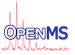OpenMS  2.8.0
FineIsotopePatternGenerator Class Reference

Isotope pattern generator for fine isotope distributions. More...

`#include <OpenMS/CHEMISTRY/ISOTOPEDISTRIBUTION/FineIsotopePatternGenerator.h>`

Inheritance diagram for FineIsotopePatternGenerator:
[legend]
Collaboration diagram for FineIsotopePatternGenerator:
[legend]

## Public Member Functions

FineIsotopePatternGenerator ()=default
Default Constructor. More...

FineIsotopePatternGenerator (double stop_condition, bool use_total_prob=true, bool absolute=false)
Constructor. More...

IsotopeDistribution run (const EmpiricalFormula &) const override
Creates an isotope distribution from an empirical sum formula. More...

void setThreshold (double stop_condition)
Set probability stop condition (lower values generate fewer results) More...

double getThreshold () const
Get probability stop condition (lower values generate fewer results) More...

void setAbsolute (bool absolute)
Set whether threshold is absolute or relative probability (ignored if use_total_prob is true, see class docu) More...

bool getAbsolute () const
Returns whether threshold is absolute or relative probability (ignored if use_total_prob is true, see class docu) More...

void setTotalProbability (bool total)
Set whether total probability should be computed. More...

bool getTotalProbability () const
Returns whether total probability should be computed. More...Public Member Functions inherited from IsotopePatternGenerator
IsotopePatternGenerator ()

IsotopePatternGenerator (double probability_cutoff)

virtual ~IsotopePatternGenerator ()

## Protected Attributes

double stop_condition_ = 0.01

bool absolute_ = false

bool use_total_prob_ = trueProtected Attributes inherited from IsotopePatternGenerator
double min_prob_

## Detailed Description

Isotope pattern generator for fine isotope distributions.

This algorithm generates theoretical pattern distributions for empirical formulas with high resolution (while the CoarseIsotopePatternGenerator will generate low-resolution patterns). The output is a list of pairs containing isotope probabilities paired with the accurate m/z for the analyte isotopic composition.

For example, for a C100H202 molecule (at 0.01 threshold), you will get:

m/z 1403.5806564438 : INT 0.333207070827484
m/z 1404.5840114438 : INT 0.360387712717056
m/z 1404.5869331919 : INT 0.00774129061028361
m/z 1405.5873664438 : INT 0.19294385612011
m/z 1405.5902881919 : INT 0.00837276969105005
m/z 1406.5907214438 : INT 0.0681697279214859
m/z 1406.5936431919 : INT 0.00448260130360723
m/z 1407.5940764438 : INT 0.0178796537220478
m/z 1407.5969981919 : INT 0.00158376491162926
...

For comparison, the CoarseIsotopePatternGenerator will produce the following result for a C100H202 molecule:

m/z 1403.58 INT: 0.333489
m/z 1404.58 INT: 0.36844
m/z 1405.59 INT: 0.201576
m/z 1406.59 INT: 0.0728113
m/z 1407.59 INT: 0.0195325
...

One important value to set is the threshold with tells the algorithm when to stop calculating isotopic peaks to calculate. The default stop condition is to stop when only a small portion (such as 0.01) of the total probability is unexplained and the reported values cover most of the probability (e.g. 0.99).

Another way to stop the search is when any new peak would be less than 0.01 in height (absolute) or when it would be less than 0.01 of the highest isotopic peak (relative). This is how the stop_condition parameter is interpreted when use_total_prob is set to false.

Note
Computation of fine isotope patterns can be slow for large molecules, if you don't need fine isotope distributions consider using CoarseIsotopePatternGenerator.
Consider using IsoSpec directly or the OpenMS IsoSpecWrapper / IsoSpecGeneratorWrapper classes defined in IsoSpecWrapper.h for increased performance.

The computation is based on the IsoSpec algorithm

Łącki MK, Startek M, Valkenborg D, Gambin A.
IsoSpec: Hyperfast Fine Structure Calculator.
Anal Chem. 2017 Mar 21;89(6):3272-3277. doi: 10.1021/acs.analchem.6b01459.

## ◆ FineIsotopePatternGenerator() [1/2]

 FineIsotopePatternGenerator ( )
default

Default Constructor.

## ◆ FineIsotopePatternGenerator() [2/2]

 FineIsotopePatternGenerator ( double stop_condition, bool use_total_prob = `true`, bool absolute = `false` )
inline

Constructor.

Parameters
 stop_condition The total probability (if use_total_prob == true) or threshold (if use_total_prob is false) (see class docu) use_total_prob Whether the stop_condition should be interpreted as a probability threshold (only configurations with intensity above this threshold will be returned) or as a total probability that the distribution should cover. absolute Whether threshold is absolute or relative (ignored if use_total_prob is true, see class docu)

## ◆ getAbsolute()

 bool getAbsolute ( ) const
inline

Returns whether threshold is absolute or relative probability (ignored if use_total_prob is true, see class docu)

## ◆ getThreshold()

 double getThreshold ( ) const
inline

Get probability stop condition (lower values generate fewer results)

## ◆ getTotalProbability()

 bool getTotalProbability ( ) const
inline

Returns whether total probability should be computed.

## ◆ run()

 IsotopeDistribution run ( const EmpiricalFormula & ) const
overridevirtual

Creates an isotope distribution from an empirical sum formula.

Iterates through all elements, convolves them according to the number of atoms from that element and sums up the result.

Note
The constructed isotope distribution is sorted by m/z which slows down processing, consider using IsoSpec (IsoSpecWrapper / IsoSpecGeneratorWrapper) directly for increased performance.

Implements IsotopePatternGenerator.

## ◆ setAbsolute()

 void setAbsolute ( bool absolute )
inline

Set whether threshold is absolute or relative probability (ignored if use_total_prob is true, see class docu)

## ◆ setThreshold()

 void setThreshold ( double stop_condition )
inline

Set probability stop condition (lower values generate fewer results)

## ◆ setTotalProbability()

 void setTotalProbability ( bool total )
inline

Set whether total probability should be computed.

## ◆ absolute_

 bool absolute_ = false
protected

## ◆ stop_condition_

 double stop_condition_ = 0.01
protected

## ◆ use_total_prob_

 bool use_total_prob_ = true
protected